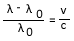## Cosmology

16.1 - Know that observations of galaxies outside the Local Group show that light is shifted to longer wavelengths (redshift)

16.2 - Understand that redshift is caused by galaxies receding from us

16.3 - Be able to use the formula:where λ is the observed wavelength, λ0 is the emitted wavelength, v is the radial velocity of the source, c is the speed of light

16.4 - Understand the evidence to confirm the discovery of the expanding universe

16.5 - Be able to use the relationship between distance and redshift of distant galaxies (Hubble’s law) including the formula:
v = H0d
where v is the radial velocity of the recession of the galaxy, H0 is the Hubble constant and d is the distance of the galaxy from Earth.

16.6 - Understand the estimation of the age and size of the Universe using the value of the Hubble constant

16.7 - Understand how the expansion of the Universe supports both the Big Bang theory and the Steady State theory

16.8 - Understand the major observational evidence in favour of the Big Bang theory:
a) quasars (QSOs)
b) cosmic microwave background (CMB) radiation
c) Hubble Deep Field image

16.9 - Understand the significance of the fluctuations in the CMB radiation for theories of the evolution of the Universe, including discoveries by the Wilkinson Microwave Anisotropy Probe (WMAP) and the Planck mission

16.10 - Understand the significance and possible nature of dark matter and dark energy

16.11 - Understand the difficulties involved in the detection of dark matter and dark energy

16.12 - Understand that current models of the Universe predict different future evolutionary paths

In this section you will learn how galaxies tell us about the age of the Universe and how the Universe came into existence.

In this section you will see the term 'cosmologists'. These are astronomers who study the Universe and cosmology.

At the end of this section take the mini quiz to test yourself.